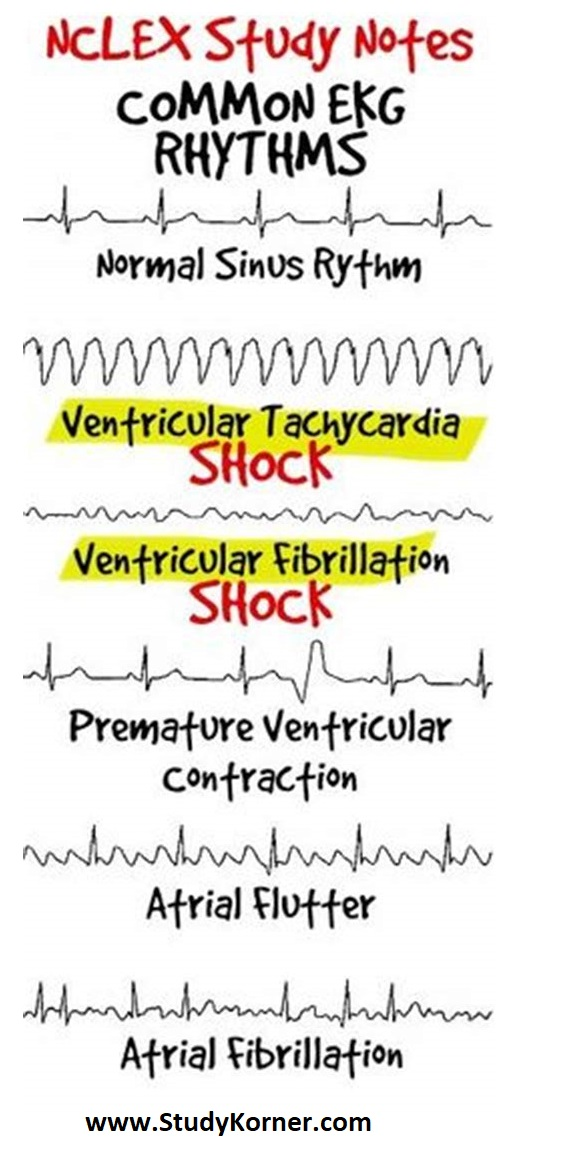# NCLEX Study Notes: Common EKG/ECG Rhythms Nursing

NCLEX Study Notes: Common EKG/ECG Rhythms

## EKG/ECG for Nursing Students: How To Read An Electrocardiogram

Following the steps below will allow ease with interpretation of arrhythmias. Analyzing strips in this order and reviewing these 5 aspects will allow for correct identification of cardiac rhythms.

Rate
P Waves
P:QRS
QRS
PR Interval

Rate
What is the ventricular rate?
On a six second strip count the R waves and multiply by 10
Alternatively, you can divide 300 by the number of large squares between R waves or P waves. This method is less reliably if the rate is irregular.

P Waves
Are there P Waves?
What is the rate of the P wave?
What is the morphology of the P wave (upward, smooth, rounded)?

P:QRS
Is there a 1:1 relationship between P wave and QRS complex?

QRS
Are there QRS complexes?
Is the QRS width 0.06 – 0.12 seconds (1.5 – 3 small boxes)?
PR Interval
Is the PRI between 0.12 – 0.20 seconds (3 – 5 small boxes)?